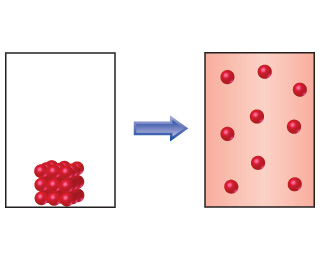Chemistry Practice Problems Entropy Practice Problems Solution: If energy can flow in and out of the system to mai...

🤓 Based on our data, we think this question is relevant for Professor Fauq's class at FSCJ.

# Solution: If energy can flow in and out of the system to maintain a constant temperature during the process, what can you say about the entropy change of the surroundings as a result of this process?

###### Problem

If energy can flow in and out of the system to maintain a constant temperature during the process, what can you say about the entropy change of the surroundings as a result of this process?###### Solution

We are asked to determine the entropy change of the surroundings as a result of the given process.Recall that entropy is the degree of chaos or disorder of a system and that it depends on the following:View Complete Written Solution

Entropy

Entropy

#### Q. For each of the following pairs, predict which substance has a higher entropy per mole at a given temperature.He(g) at 5 atm pressure or He(g) at 1.8 ...

Solved • Thu Jan 03 2019 09:45:16 GMT-0500 (EST)

Entropy

#### Q. If the normal boiling point of a liquid is 67 oC, and the standard molar entropy change for the boiling process is +100 J/K, estimate the standard mol...

Solved • Thu Jan 03 2019 09:45:14 GMT-0500 (EST)

Entropy

#### Q. Indicate whether each statement is true or false.Unlike enthalpy, where we can only ever know changes in H, we can know absolute values of S.

Solved • Thu Jan 03 2019 09:45:10 GMT-0500 (EST)

Entropy

#### Q. Does the entropy of the system increase, decrease, or stay the same for each of the following conditions.

Solved • Thu Jan 03 2019 09:44:56 GMT-0500 (EST)